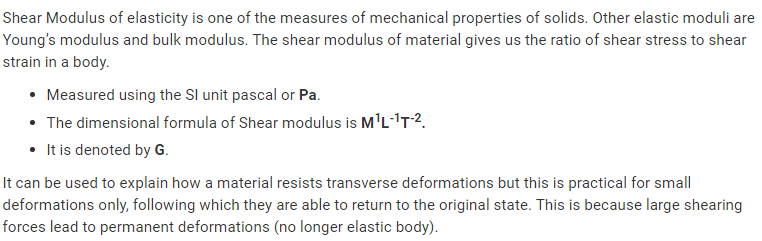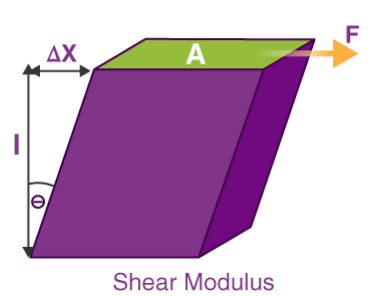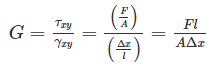# Fluids mechanics notes

### Detail fluids mechanics notes

1.(a) Hooke’s law
(i) The force developed within a body is directly proportional to the deformation of the body within the elastic limit.
(ii) Force constant of a spring is the force required to produce unit extension in it.

(b) Young’s modulus

Young’s modulus is also known as modulus of elasticity and is defined as:

The mechanical property of a material to withstand the compression or the elongation with respect to its length.

It is denoted as E or Y.

Young’s Modulus (also referred to as the Elastic Modulus or Tensile Modulus), is a measure of mechanical properties of linear elastic solids like rods, wires, and such. There are other numbers that give us a measure of elastic properties of a material, like Bulk modulus and shear modulus, but the value of Young’s Modulus is most commonly used. This is because it gives us information about the tensile elasticity of a material (ability to deform along an axis).

Young’s modulus describes the relationship between stress (force per unit area) and strain (proportional deformation in an object). The Young’s modulus is named after the British scientist Thomas Young. A solid object deforms when a particular load is applied to it. If the object is elastic, the body regains its original shape when the pressure is removed. Many materials are not linear and elastic beyond a small amount of deformation. The constant Young’s modulus applies only to linear elastic substances.

Young's Modulus Formula: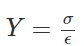Bulk modulus of Elasticity: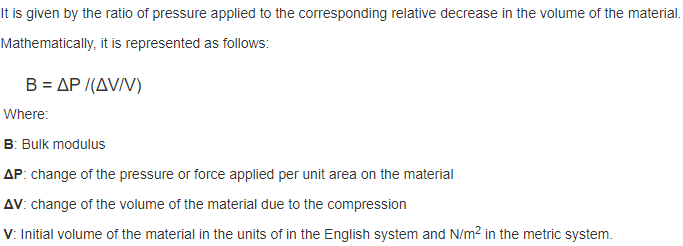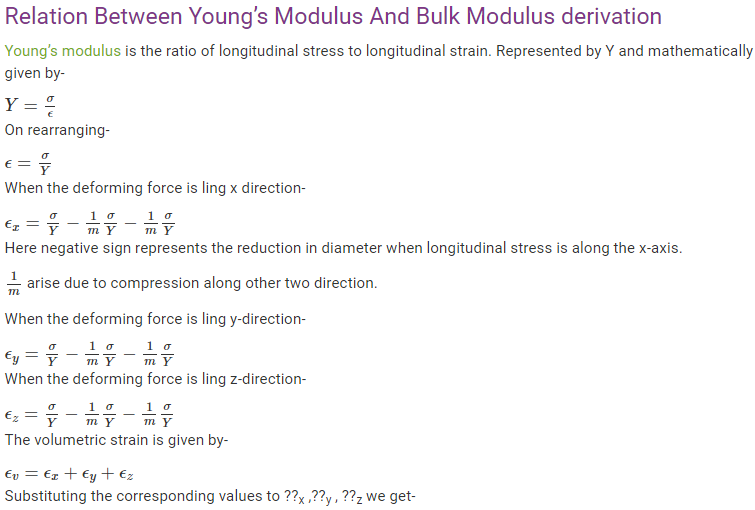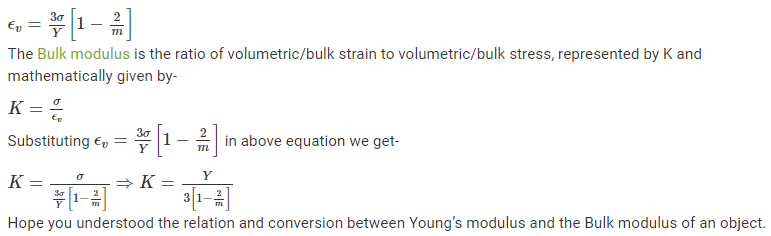Shear Modulus of Rigidity: